# 2015-06-15 Large-Scale Elastic-Net Regularized Generalized Linear Models at Spark Summit 2015

Jun. 16, 2015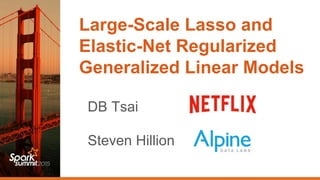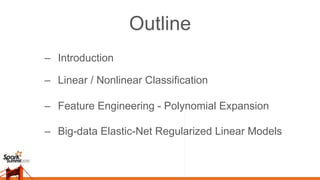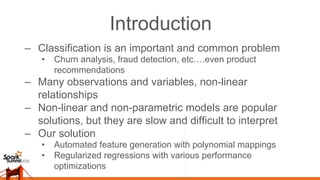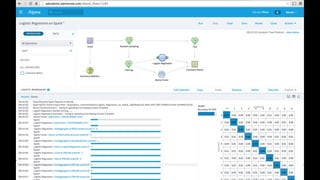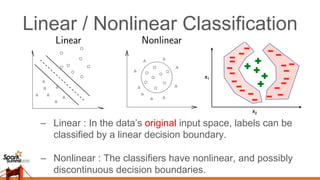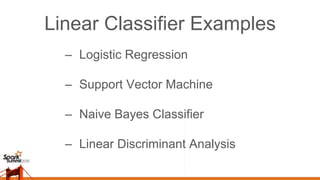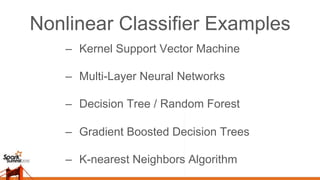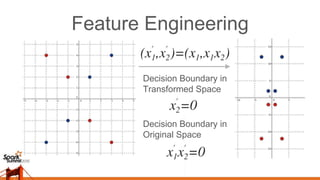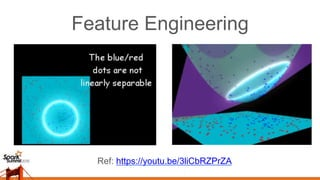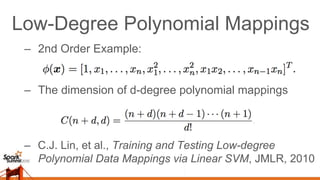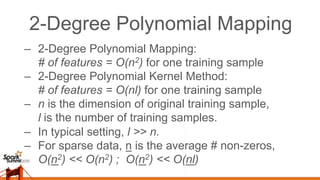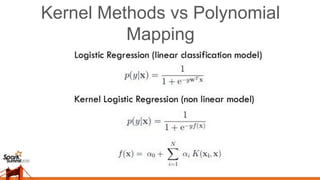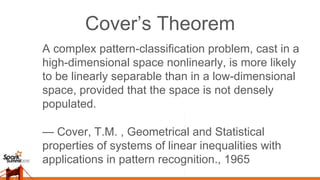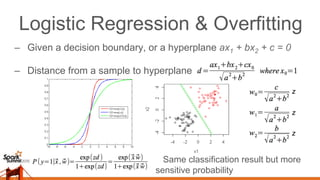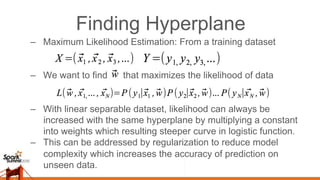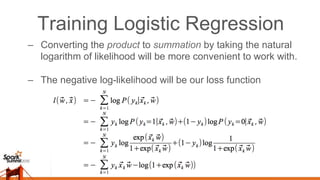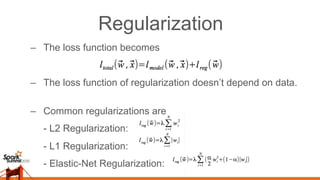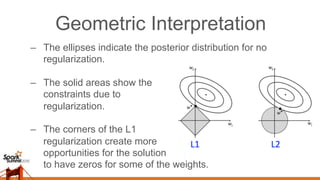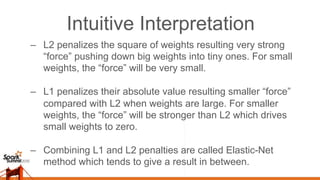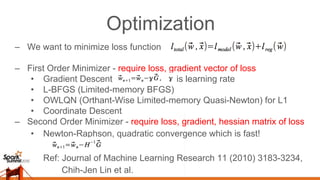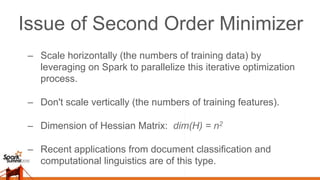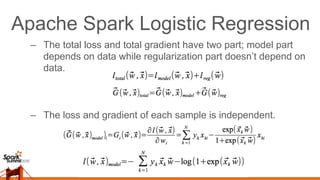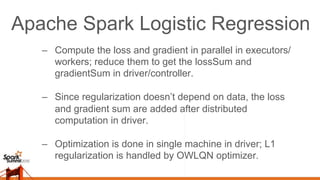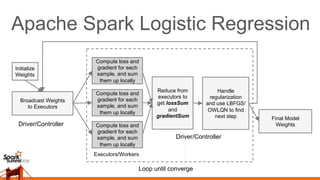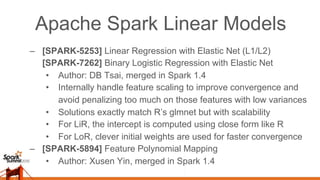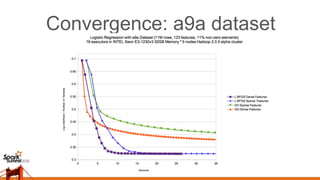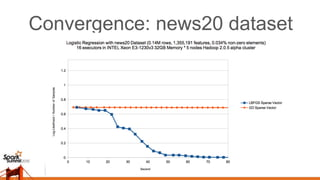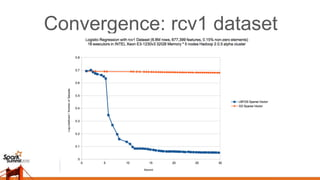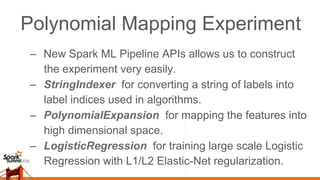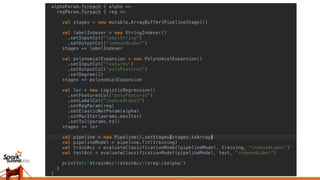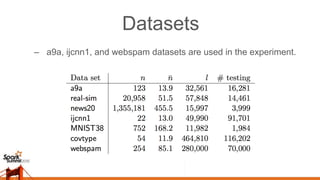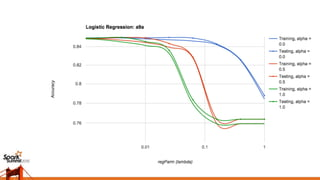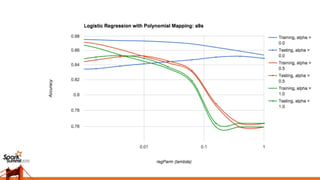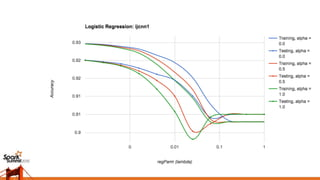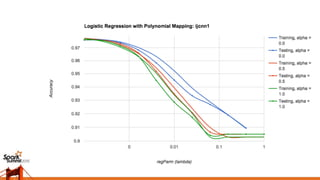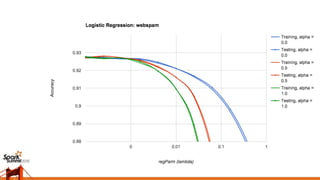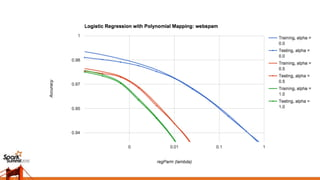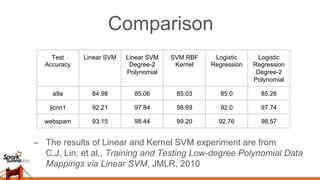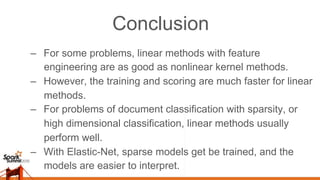1 of 40

### 2015-06-15 Large-Scale Elastic-Net Regularized Generalized Linear Models at Spark Summit 2015

• 1. Large-Scale Lasso and Elastic-Net Regularized Generalized Linear Models DB Tsai Steven Hillion
• 2. Outline –  Introduction –  Linear / Nonlinear Classification –  Feature Engineering - Polynomial Expansion –  Big-data Elastic-Net Regularized Linear Models
• 3. Introduction –  Classification is an important and common problem •  Churn analysis, fraud detection, etc….even product recommendations –  Many observations and variables, non-linear relationships –  Non-linear and non-parametric models are popular solutions, but they are slow and difficult to interpret –  Our solution •  Automated feature generation with polynomial mappings •  Regularized regressions with various performance optimizations
• 5. –  Linear : In the data’s original input space, labels can be classified by a linear decision boundary. –  Nonlinear : The classifiers have nonlinear, and possibly discontinuous decision boundaries. Linear / Nonlinear Classification
• 6. Linear Classifier Examples –  Logistic Regression –  Support Vector Machine –  Naive Bayes Classifier –  Linear Discriminant Analysis
• 7. Nonlinear Classifier Examples –  Kernel Support Vector Machine –  Multi-Layer Neural Networks –  Decision Tree / Random Forest –  Gradient Boosted Decision Trees –  K-nearest Neighbors Algorithm
• 8. Feature Engineering Decision Boundary in Transformed Space Decision Boundary in Original Space
• 9. Feature Engineering Ref: https://youtu.be/3liCbRZPrZA
• 10. Low-Degree Polynomial Mappings –  2nd Order Example: –  The dimension of d-degree polynomial mappings –  C.J. Lin, et al., Training and Testing Low-degree Polynomial Data Mappings via Linear SVM, JMLR, 2010
• 11. 2-Degree Polynomial Mapping –  2-Degree Polynomial Mapping: # of features = O(n2) for one training sample –  2-Degree Polynomial Kernel Method: # of features = O(nl) for one training sample –  n is the dimension of original training sample, l is the number of training samples. –  In typical setting, l >> n. –  For sparse data, n is the average # non-zeros, O(n2) << O(n2) ; O(n2) << O(nl)
• 12. Kernel Methods vs Polynomial Mapping
• 13. Cover’s Theorem A complex pattern-classification problem, cast in a high-dimensional space nonlinearly, is more likely to be linearly separable than in a low-dimensional space, provided that the space is not densely populated. — Cover, T.M. , Geometrical and Statistical properties of systems of linear inequalities with applications in pattern recognition., 1965
• 14. Logistic Regression & Overfitting –  Given a decision boundary, or a hyperplane ax1 + bx2 + c = 0 –  Distance from a sample to hyperplane Same classification result but more sensitive probability z z z
• 15. Finding Hyperplane –  Maximum Likelihood Estimation: From a training dataset –  We want to find that maximizes the likelihood of data –  With linear separable dataset, likelihood can always be increased with the same hyperplane by multiplying a constant into weights which resulting steeper curve in logistic function. –  This can be addressed by regularization to reduce model complexity which increases the accuracy of prediction on unseen data.
• 16. Training Logistic Regression –  Converting the product to summation by taking the natural logarithm of likelihood will be more convenient to work with. –  The negative log-likelihood will be our loss function
• 17. Regularization –  The loss function becomes –  The loss function of regularization doesn’t depend on data. –  Common regularizations are - L2 Regularization: - L1 Regularization: - Elastic-Net Regularization:
• 18. Geometric Interpretation –  The ellipses indicate the posterior distribution for no regularization. –  The solid areas show the constraints due to regularization. –  The corners of the L1 regularization create more opportunities for the solution to have zeros for some of the weights.
• 19. Intuitive Interpretation –  L2 penalizes the square of weights resulting very strong “force” pushing down big weights into tiny ones. For small weights, the “force” will be very small. –  L1 penalizes their absolute value resulting smaller “force” compared with L2 when weights are large. For smaller weights, the “force” will be stronger than L2 which drives small weights to zero. –  Combining L1 and L2 penalties are called Elastic-Net method which tends to give a result in between.
• 20. Optimization –  We want to minimize loss function –  First Order Minimizer - require loss, gradient vector of loss •  Gradient Descent is learning rate •  L-BFGS (Limited-memory BFGS) •  OWLQN (Orthant-Wise Limited-memory Quasi-Newton) for L1 •  Coordinate Descent –  Second Order Minimizer - require loss, gradient, hessian matrix of loss •  Newton-Raphson, quadratic convergence which is fast! Ref: Journal of Machine Learning Research 11 (2010) 3183-3234, Chih-Jen Lin et al.
• 21. Issue of Second Order Minimizer –  Scale horizontally (the numbers of training data) by leveraging on Spark to parallelize this iterative optimization process. –  Don't scale vertically (the numbers of training features). –  Dimension of Hessian Matrix: dim(H) = n2 –  Recent applications from document classification and computational linguistics are of this type.
• 22. Apache Spark Logistic Regression –  The total loss and total gradient have two part; model part depends on data while regularization part doesn’t depend on data. –  The loss and gradient of each sample is independent.
• 23. Apache Spark Logistic Regression –  Compute the loss and gradient in parallel in executors/ workers; reduce them to get the lossSum and gradientSum in driver/controller. –  Since regularization doesn’t depend on data, the loss and gradient sum are added after distributed computation in driver. –  Optimization is done in single machine in driver; L1 regularization is handled by OWLQN optimizer.
• 24. Apache Spark Logistic Regression Broadcast Weights to Executors Driver/Controller Executors/Workers Loop until converge Initialize Weights Compute loss and gradient for each sample, and sum them up locally Final Model Weights Compute loss and gradient for each sample, and sum them up locally Compute loss and gradient for each sample, and sum them up locally Reduce from executors to get lossSum and gradientSum Handle regularization and use LBFGS/ OWLQN to find next step Driver/Controller
• 25. Apache Spark Linear Models –  [SPARK-5253] Linear Regression with Elastic Net (L1/L2) [SPARK-7262] Binary Logistic Regression with Elastic Net •  Author: DB Tsai, merged in Spark 1.4 •  Internally handle feature scaling to improve convergence and avoid penalizing too much on those features with low variances •  Solutions exactly match R’s glmnet but with scalability •  For LiR, the intercept is computed using close form like R •  For LoR, clever initial weights are used for faster convergence –  [SPARK-5894] Feature Polynomial Mapping •  Author: Xusen Yin, merged in Spark 1.4
• 26. Convergence: a9a dataset
• 27. Convergence: news20 dataset
• 28. Convergence: rcv1 dataset
• 29. Polynomial Mapping Experiment –  New Spark ML Pipeline APIs allows us to construct the experiment very easily. –  StringIndexer for converting a string of labels into label indices used in algorithms. –  PolynomialExpansion for mapping the features into high dimensional space. –  LogisticRegression for training large scale Logistic Regression with L1/L2 Elastic-Net regularization.
• 31. Datasets –  a9a, ijcnn1, and webspam datasets are used in the experiment.
• 38. Comparison Test Accuracy Linear SVM Linear SVM Degree-2 Polynomial SVM RBF Kernel Logistic Regression Logistic Regression Degree-2 Polynomial a9a 84.98 85.06 85.03 85.0 85.26 ijcnn1 92.21 97.84 98.69 92.0 97.74 webspam 93.15 98.44 99.20 92.76 98.57 –  The results of Linear and Kernel SVM experiment are from C.J. Lin, et al., Training and Testing Low-degree Polynomial Data Mappings via Linear SVM, JMLR, 2010
• 39. Conclusion –  For some problems, linear methods with feature engineering are as good as nonlinear kernel methods. –  However, the training and scoring are much faster for linear methods. –  For problems of document classification with sparsity, or high dimensional classification, linear methods usually perform well. –  With Elastic-Net, sparse models get be trained, and the models are easier to interpret.
• 40. Thank you! Questions?